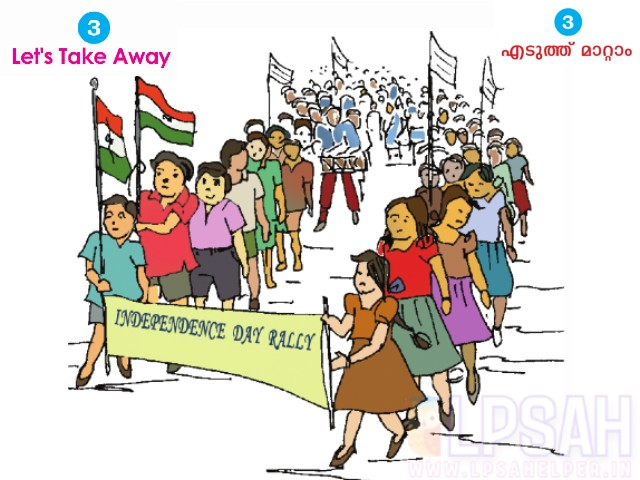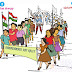LSS Examination 2022 Solved Question Paper :- ]><[

## RELATED POSTSIn the rally conducted by Scouts and Guides and the Red-Cross, 296 boys and 336 girls participated. Of this, 186 were Scouts and 177 were Guides. Then, how many were from Red - Cross?
Number of Boys = 296
Number of Girls = 336
Total = 336 + 296 = 632

Number of Guides = 177
Number of Scouts = 186
Total = 177 + 186 = 363

Members in Red-Cross = 632 - 363 = 269Share it: